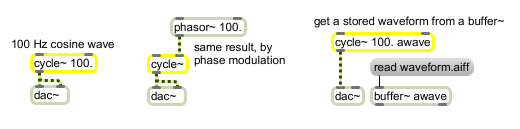# cycle~

Table lookup oscillator

## Description

The cycle~ object is an interpolating oscillator that reads repeatedly through one cycle of a waveform, using a wavetable of 512 samples. Its default waveform is one cycle of a cosine wave. It can use other waveforms by accessing samples from a buffer~ object. The 513th sample in the wavetable source (the buffer~) is used for interpolation beyond the 512th sample. For repeating waves, it's usually desirable for the 513th sample to be the same as the first sample, so there will be no discontinuity when the waveform wraps around from the end to the beginning. If only 512 samples are available, cycle~ assumes a 513th sample equal to the 1st sample.This is the case for the cycle~ object's default cosine waveform. If this is what you want for other waveforms, you should make the 513th sample the same as the 512th sample, or omit the 513th sample.

## Arguments

Name Type Opt Description
initial-frequency (Hz/cycles-per-second) float or int opt The initial frequency of the oscillator. If no frequency argument is present, the initial frequency is 0.
buffer-object-name symbol opt The name of a buffer~ object used to store the oscillator's wavetable. If a float or int frequency argument is present, the buffer~ name follows the frequency. (No frequency argument is required, however.) If no buffer~ name is given, cycle~ uses a stored cosine wave.
sample-offset int opt If a buffer~ name has been given, an additional final argument can used to specify the sample offset into the named buffer~ object's sample memory. cycle~ only uses the first channel of a multi-channel buffer~.

## Messages

 int frequency (Hz/cycles-per-second) [int] In left inlet: Sets the frequency of the oscillator. If there is a signal connected to the left inlet, this number is ignored. float frequency (Hz/cycles-per-second) [float] In left inlet: Sets the frequency of the oscillator. If there is a signal connected to the left inlet, this number is ignored. (mouse) When the cycle~ object is used as a buffer, double-clicking on buffer~ opens a display window where you can view the contents of the buffer~. object that it references. set buffer-object-name [symbol]sample-offset [int] The word set, followed by the name of a buffer~ object, changes the wavetable used by cycle~. The name can optionally be followed by an int specifying the sample offset into the named buffer~ object's sample memory. cycle~ uses only the first (left) channel of a multi-channel buffer~. The word set with no arguments reverts cycle~ to the use of its default cosine wave. signal In left inlet: Frequency of the oscillator. Negative values are allowed. In right inlet: Phase, expressed as a fraction of a cycle, from 0 to 1. Other values are wrapped around to stay in the 0 to 1 range. If the frequency is 0, connecting a phasor~ to this inlet is an alternative method of producing an oscillator. If the frequency is non-zero, connecting a cycle~ or other repeating function to this inlet produces phase modulation, which is similar to frequency modulation.

## Output

signal: A waveform (cosine by default) repeating at the specified frequency, with the specified phase.

## ExamplesRepeated cosine or any other waveform

Name Description
buffer~ Store audio samples
buffir~ buffer-based FIR filter
cos~ Signal cosine function (0-1 range)
line~ Linear ramp generator
phasor~ Sawtooth wave generator
rect~ Antialiased rectangular (pulse) oscillator
saw~ Antialiased sawtooth oscillator
techno~ Signal-driven step sequencer
trapezoid~ Trapezoidal wavetable
tri~ Antialiased triangular oscillator
triangle~ Triangle/ramp wavetable
wave~ Variable size wavetable
2d.wave~ Two-dimensional wavetable